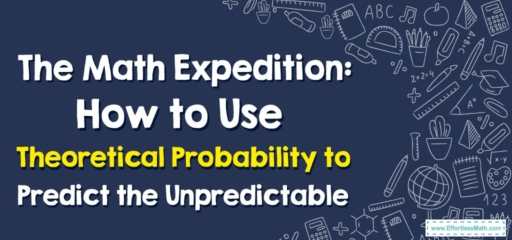# The Math Expedition: How to Use Theoretical Probability to Predict the Unpredictable

Greetings, fellow math explorers!

As we set our compass toward the fascinating world of theoretical probability today, we aim to learn how this mathematical concept can help us make predictions. So, strap on your adventure gear, and let's set forth on this thrilling journey!## 1. The Summit of Understanding: Theoretical Probability

Perched high in the mountain range of mathematics is the peak of theoretical probability – the mathematical calculation that an event will happen based on the total number of equally likely outcomes.

## 2. The Trek: Making Predictions with Theoretical Probability

Our trek today involves using theoretical probability to make predictions. Here’s our map for the journey:

### The Explorer’s Map: Using Theoretical Probability to Make Predictions

#### Step 1: Understand the Event

Our journey begins with understanding the event. What are we trying to predict?

#### Step 2: Calculate Theoretical Probability

Next, we calculate the theoretical probability of the event occurring. This is done by dividing the number of desired outcomes by the total number of outcomes.

#### Step 3: Make a Prediction

Finally, we use our calculated theoretical probability to make a prediction about the likelihood of the event happening.

Let’s take an example. Suppose we’re predicting the chance of rolling a $$3$$ on a standard six-sided die.

1. Understand the Event: We want to find out the chance of rolling a $$3$$.
2. Calculate Theoretical Probability: There’s only one $$3$$ on the die, and there are six possible outcomes $$(1, 2, 3, 4, 5, 6)$$. So, the theoretical probability of rolling a $$3$$ is $$1$$ (desired outcome) divided by $$6$$ (total outcomes), which is approximately $$0.1667$$ or $$16.67$$$$\%$$.
3. Make a Prediction: Based on our calculated theoretical probability, we might predict that there is a $$16.67$$$$\%$$ chance of rolling a $$3$$.

And with that, we’ve reached the summit of our mathematical expedition! Remember, theoretical probability is a powerful tool in your explorer’s toolkit, helping you predict the unpredictable. Until our next adventure, stay curious and keep exploring!

### What people say about "The Math Expedition: How to Use Theoretical Probability to Predict the Unpredictable - Effortless Math: We Help Students Learn to LOVE Mathematics"?

No one replied yet.

X
30% OFF

Limited time only!

Save Over 30%

SAVE $5 It was$16.99 now it is \$11.99